# Servo Motor Tutorial for Arduino, ESP8266 and ESP32

In this tutorial you learn everything you need to know about the functionalities of a servomotor.

First we learn the theoretical foundations how a servomotor works.

In multiple example you learn how to control one or multiple servo with your Arduino, ESP8266 or ESP32 microcontroller.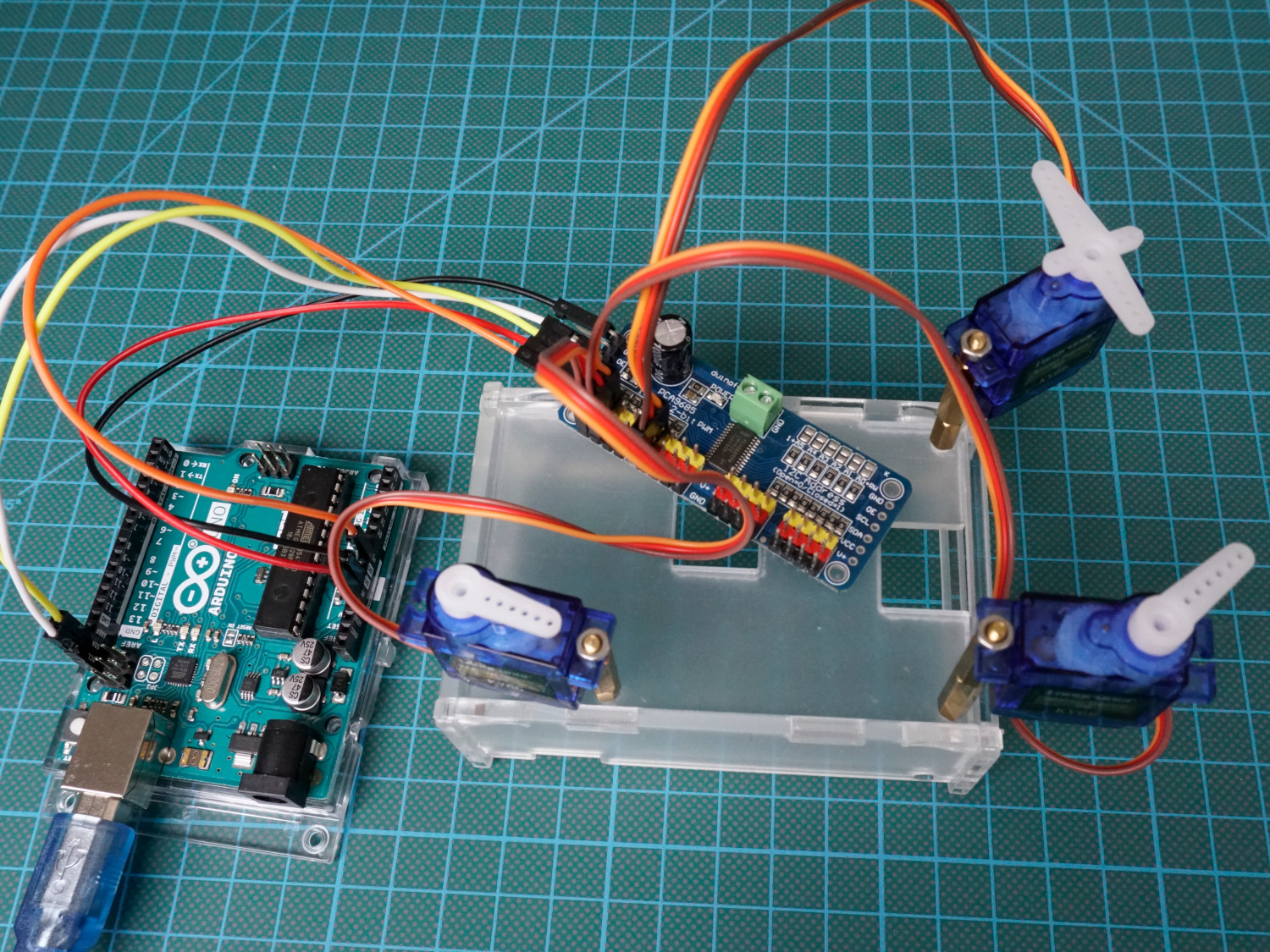A servomotor is an electric motor which can precisely control the angle position, velocity and acceleration. Therefore the motor is using a closed-loop feedback mechanism. A Servo consists of the following parts:

• An electric motor (DC motor, asynchronous motor or synchronous motor)
• A sensor for position feedback
• A controller to regulate the movement of the motor according to one or more adjustable setpoints, such as the desired angular position of the shaft or setpoint speed, in a control loop.

Servos are used in applications like robotics, CNC machines and different fields of automation technology.

## Overview of Closed-Loop Servomechanism

As mentioned at the beginning of this tutorial the servomotor uses a closed-loop feedback system to control the angle position, velocity and acceleration. The following picture shows the closed-loop.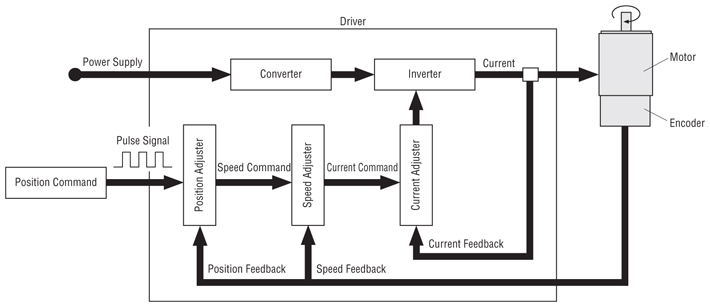### Forward Path in Closed-Loop Servomechanism

The input for the driver is a pulse signal, which will be provided by our microcontroller in the following examples of this tutorial. The pulse signal define the position adjustment for the servo which results in a speed command and final in a current command which is the input for the inverter to turn the motor in the preferred direction.

There are different types of motors that can be used for a servo because the servomotor is not depended on the motor but on the functionality with the closed feedback loop. The most used motors are from brushed permanent magnet DC motors which are simple and cheap to AC induction motors for industrial use.

### Backward Path in Closed-Loop Servomechanism

The motor is paired with an encoder to provide speed and position feedback. The current feedback is provided directly by the inverter.

In out example we use a cheap servo motor which only uses the position feedback via a potentiometer. Therefore the motor always rotates at full speed. For industrial uses servomotors have a speed feedback loop as well through an optical rotary encoder. These types of servo are able to control the speed and therefore reach the desired position faster and precisely with less overshotting.

The position feedback to control the motion and the final position is compared to input signal. If there is a difference, an error signal is generated. The error signal causes the servo to rotate in the preferred direction until the error signal is zero. If the error signal is zero, the final position is reached and the motor stops rotating.

### Input Pulse Signal in Closed-Loop Servomechanism

Now we want to control the servomotor with our Arduino, ESP8266 or ESP32 microcontroller. Therefore the pulse signal as input has to be created from the microcontroller as a square wave similar to a PWM signal.

Because we do not create a PWM signal, the digital pin for the motor does not have to be a PWM pin on your board. Every digital pin will work.

Most servo motors especially the cheaper ones which I use are not able to turn full 360 degrees but rather are able to turn between 0 and 180 degrees. With the PWM signal, the control is

• 0 degrees for a pulse width of 1 ms,
• 90 degrees with a pulse width of 1.5 ms and
• 180 degrees with a 2 ms pulse width.

The total cycle time is 20 ms where the PWM is repeated. The following picture shows the three different positions with their pulse with.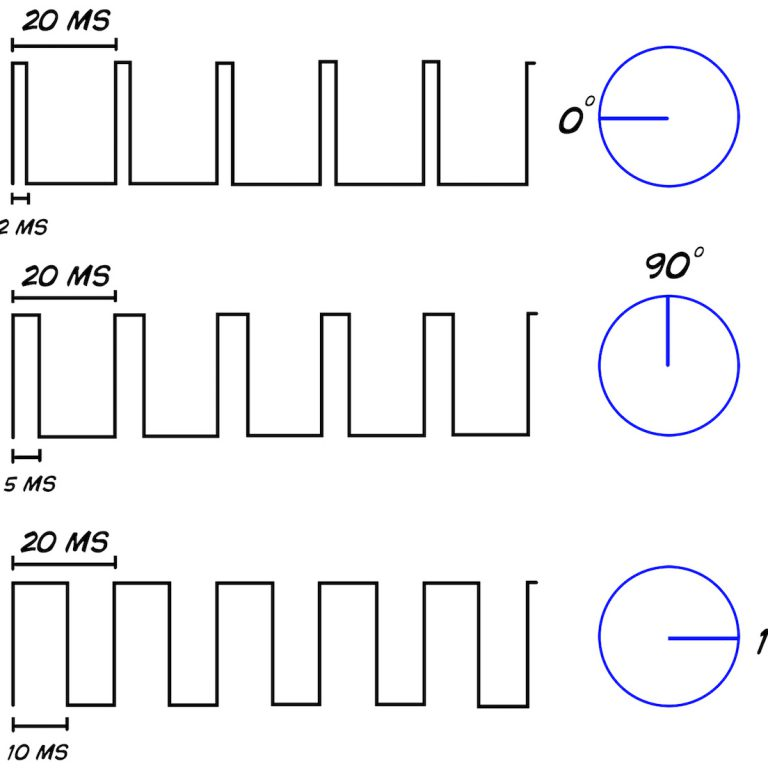## Datasheet of Longruner SG90 Micro Servo Motor 9G RC (KY66-5)

The servo I use is the Longruner SG90 Micro Servo Motor 9G RC (KY66-5). The following table shows the data sheet for the motor.

CriteriaSpecification
Operating speed0.12second/ 60degree ( 4.8V no load)
Stall Torque (4.8V)17.5oz /in (1kg/cm)
Operating voltage3.0V~7.2V
Temperature range-30 to +60 degree Celsius
Working Frequency50Hz
Motor TypeBrushed DC Motor
Gear TypePlastic Gears
Dimensions23 x 11.5 x 24mm
Weight9 grams

The stall torque is the torque the motor is pushing against a difference to the current position if you want to spin the motor with your hand. With a wide range of operating voltage the servo is able to work with Arduino boards (5V supply voltage) and EPS8266 boards (3.3V supply voltage).

The following table gives you an overview of all components and parts that I used for this tutorial. I get commissions for purchases made through links in this table.

Arduino Nano AmazonAliExpress
Arduino Pro Mini AmazonAliExpress
Arduino Uno AmazonAliExpress
Arduino Mega AmazonAliExpress
ESP32 ESP-WROOM-32AmazonAliExpress
ESP8266 NodeMCU AmazonAliExpress
ESP8266 WeMos D1 Mini AmazonAliExpress
Potentiometer / Rotary Encoder AmazonAliExpress
Servo Motor AmazonAliExpress
PCA9685 16 CH 12Bit PWM Servo Motor Driver AmazonAliExpress

We will use the Servo library in the following examples. If you do not know how to install a library in the Arduino IDE, here is a tutorial.

If we use the Servo library the PWM functionality on pin 9 and pin 10 of the Arduino boards are automatically disabled.

## How to Turn a Servo Motor up to 180 Degrees

The first example is the easiest one you can image. We want to turn the motor from 0 to 180 degrees in a step size of 1 and after pausing at 180 degrees for one second we want to turn the motor backwards to 0 degree but with a faster step size of 5.

### Wiring between Servo Motor and Microcontroller to Turn Servo 180 Degrees

The following pictures show the wiring between the Arduino, ESP8266 or ESP32 microcontroller and the servo motor.

The power supply for the servo motor is either the 5V pin for the Arduino boards or the 3.3V pin for the ESP8266 or ESP32 boards. If you power the microcontroller boards via USB, you can also use the 5V output of the ESP8266 or ESP32 boards.

To control the servo motor, we use a digital I/O pin like 9 (Arduino), D7 (ESP8266) or 4 (ESP32). You can use any other digital pin of your microcontroller, but then you must also change the program script.

For more information about the Arduino Nano, visit the Arduino Nano Tutorial.

For more information about the Arduino Uno, visit the Arduino Uno Tutorial.

For more information about the Arduino Mega, visit the Arduino Nano Mega.

### Program Script to Turn Servo 180 Degrees

After the wiring between the microcontroller and the servo motor, we create the Arduino script that moves the servo motor forward to a degree of 180 and back to 0 degree. I describe the script that is written for Arduino, ESP8266 and ESP32 microcontroller boards in detail.

```#include "Servo.h"

int servo_pin = 9;  // for Arduino microcontroller
//int servo_pin = D7;  // for ESP8266 microcontroller
//int servo_pin = 4;  // for ESP32 microcontroller

Servo myservo;
int angle = 0;

void setup()
{
myservo.attach(servo_pin);
}

void loop()
{ // move from 0 to 180 degrees with a positive angle of 1
for(angle = 0; angle < 180; angle += 1)
{
myservo.write(angle);
delay(15);
}

delay(1000);

// move from 180 to 0 degrees with a negative angle of 5
for(angle = 180; angle>=1; angle-=5)
{
myservo.write(angle);
delay(5);
}

delay(1000);
}```

At the beginning of the script we include the Servo library that makes the operation of the servo motor a lot easier.

Then we have to define the digital I/O pin that connects the microcontroller to the servo motor. Because the used pin depends on the microcontroller, I created in total 3 lines of code that matches the wiring pictures before. Dependent on your microcontroller you have to comment 2 lines and uncomment the line of code that matches your microcontroller.

Now we can initialize a servo object from the previous included servo library.

Out objective is to rotate the servo to a certain degree in each loop of the program code. Therefore we need a variable to store the current angle of the motor. The initial value of the angle is 0.

In the setup function we use the attach function of the servo object to tell the object, that the servo motor is connected to the servo pin.

In the loop function we need two for loops to turn the motor from 0 degree to 180 degrees and a second function that moves the motor back to 0 degree but with another speed.

Therefore in each for loop we use the write function of the myservo object to define the absolute angle that the servo should turn. This angle can be positive and also negative.

In the first for loop we increase the angle each loop by +1 and use a delay of 15 milliseconds. Therefore the motor turns relative slow.

In the second loop function, where we want to move the servo back to 0 degree, we decrease the angle every loop by 5 and use a delay of 5 milliseconds. The motor turns faster from 180 degrees to 0 degree.

At the end of the script we wait for 1 second and start the loop function all over again.

The following video, shows the Arduino script in action with an Arduino Uno as example.## Set the Servo Motor to a specific Angle with a Potentiometer

In the second example we want to use a potentiometer to set the servo motor to a specific angle. Therefore we read the value of the potentiometer which is between 0 and 1023, re-scale the value between 0 and 180 to move the motor between 0 and 180 degrees.

### Wiring between the Servo Motor, Microcontroller and Potentiometer

The following picture show the wiring between the Arduino, EPS8266 or ESP32 microcontroller board, the servo motor and the potentiometer.

The wiring is based on the first wiring where we only use the servo motor and the microcontroller. Now we have to add the connection for the potentiometer. We use the same pins for the power supply and have to use a analog pin to read the current analog value of the potentiometer.

For more information about the Arduino Nano, visit the Arduino Nano Tutorial.

For more information about the Arduino Uno, visit the Arduino Uno Tutorial.

For more information about the Arduino Mega, visit the Arduino Mega Tutorial.

### Program Code to Set the Servo Motor to a specific Angle

The following section shows and explains the Arduino program code for Arduino, ESP8266 and ESP32 microcontroller to set the servo motor to a specific angle with the input of a potentiometer.

```#include "Servo.h"

// for Arduino microcontroller
int servo_pin = 9;
int potpin = 0;

// for ESP8266 microcontroller
//int servo_pin = D7;
//int potpin = A0;

// for ESP32 microcontroller
//int servo_pin = 4;
//int potpin = 0;

Servo myservo;
int val;

void setup() {
myservo.attach(servo_pin);
}

void loop() {
val = map(val, 0, 1023, 0, 180);
myservo.write(val);
delay(15);
}```

The first part of the Arduino script is very similar to the previous one. At the start we have to include the servo library and define the pins that connect the microcontroller board with the servo. Because we have another connected component in this example, we also have to define the analog pin that connects the potentiometer and the Arduino, ESP8266 or ESP32 board.

If you are not sure which pins of the Arduino, ESP8266 or ESP32 microcontroller board can be used to connect different electrical components, I can recommend the Microcontroller Datasheet eBook.

After we initialize a servo object, we create an variabel to store the value of the potentiometer that is between 0 and 1023.

The setup function stays the same, we only have to tell the servo object at which pin the motor is connected.

In the loop function, we read the analog value of the potentiometer and map the value range of the potentiometer (0…1023) to the value range of the servo (0…180). After the mapping of the values, we set the angle of the servo to the mapped value of the potentiometer with the write function and include a short delay of 15 milliseconds to make sure that the servo is able to rotate in the desired position.

The following video shows how the servo is rotating, controlled by the potentiometer.Microcontroller Datasheet eBook

The 35 pages Microcontroller Datasheet Playbook contains the most useful information of 14 Arduino, ESP8266 and ESP32 microcontroller boards.

## Connect Multiple Servo Motors

Of cause it is possible to connect multiple servos to your microcontroller and the Arduino Mega has a lot of pins. But there is a smarter way to connect multiple servos to your Arduino or NodeMCU using the I2C connection. If you want to know more about the I2C connection, you find a complete guide in this article.

### PCA9685 16-Channel Servo Driver

To connect multiple servo motors over I2C we use the PCA9685 16-Channel Servo Driver which uses an on-board PWM controller to drive all 16 channels at the same time. Additionally you are able to connect multiple PCA9685 16-Channel Servo Drivers in a chain up to 62 drivers. Therefore we are able to control up to 992 servos with the 2 I2C pins.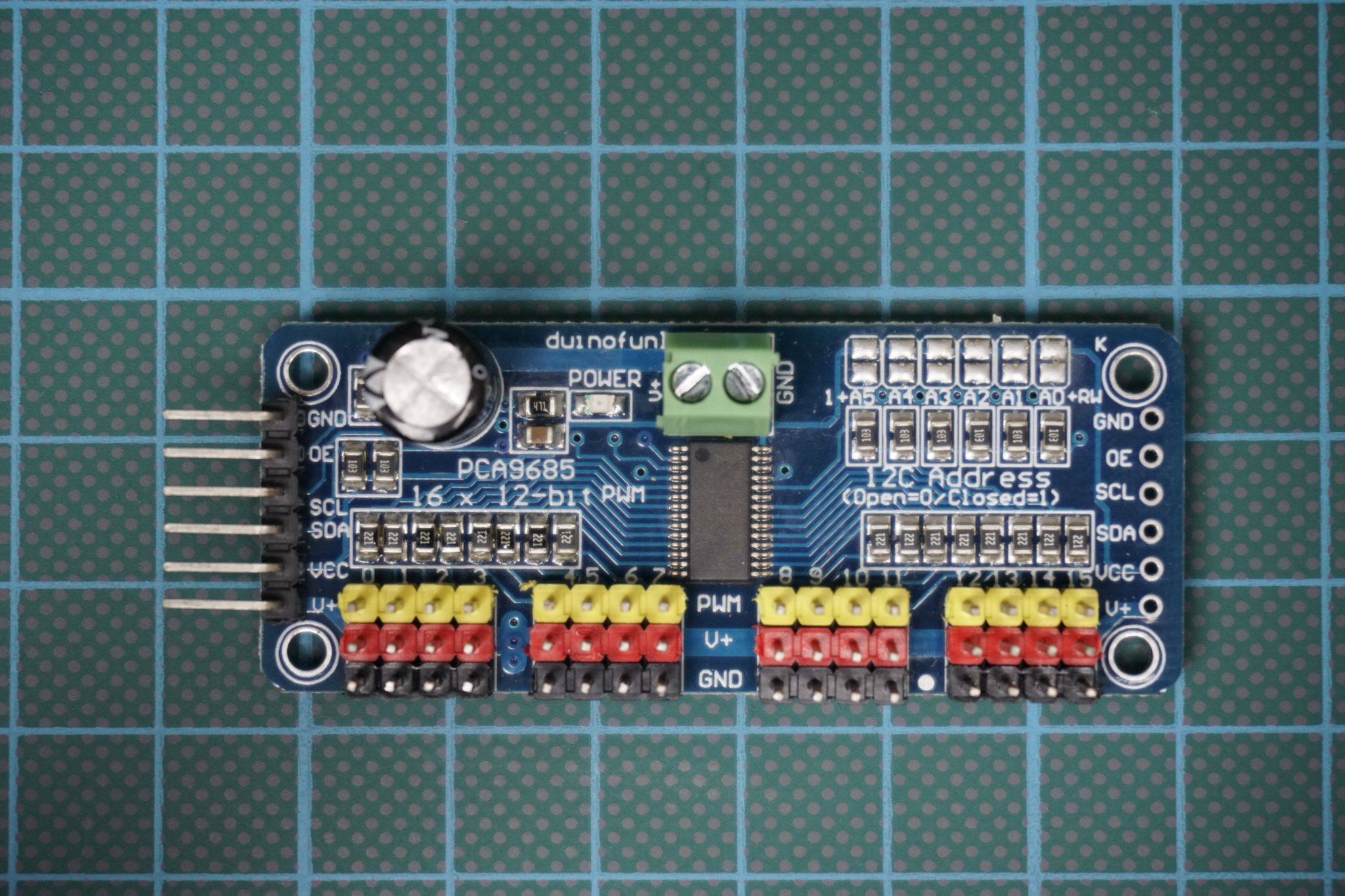The connection between the PCA9685 and the microcontroller is easy and shown in the following table as well as in the fritzing sketch.

PCA9685DescriptionArduinoESP32ESP8266
GNDGroundGNDGNDGND
VCCLogic power pin which should be between 3V and 5V5V3.3V3.3V
V+Optional power pin to supply the servo motors. Either connect V+ to VIN or use the 2-pin terminal block at the top of the PCA9685 board. Provide 5V DC to 12V DC. Note: Do not connect V+ and the 2-pin terminal at the same time.VINVIN
SCLI2C clock pinSCL pin (19)SCL pin (22)SCL pin (D1)
SDAI2C data pinSDA pin (18)SDA pin (21)SDA pin (D2)
OECan be used to quickly disable all output when pulled HIGH. We do not use the functionality

If you do not know the SCL and SDA pins for your microcontroller, you find the pinouts for each board in the following articles: Arduino Nano, Arduino Uno, Arduino Mega, ESP8266, ESP32 or better in the Microcontroller Datasheet Playbook.

The 16 output ports (V+, GND and PWM) can be used to connect servos or LEDs. All outputs use the same PWM frequency which can be 1 kHz for LEDs and 60 Hz for servos. The maximum current per pin is 25mA. If you only use some servos like up to five you can power them from your microcontroller. But if you want to use more motors, I would recommend to power the servos with the 2-pin terminal and an external power supply. In the list of the parts you find some good choices for an external power supply.

### Wiring between the PCA9685 and the Microcontroller

The following pictures shows the wiring when we do not use an external power source for the PCA9685 board.

For more information about the Arduino Nano, visit the Arduino Nano Tutorial.

For more information about the Arduino Uno, visit the Arduino Uno Tutorial.

For more information about the Arduino Mega, visit the Arduino Mega Tutorial.

And if we want to use a lot of servo motors and and therefore need an external power supply, we have to disconnect the connection between V+ and the microcontroller and therefore connect the PCA9685 to an external power supply between 5V and 12V.

For more information about the Arduino Nano, visit the Arduino Nano Tutorial.

For more information about the Arduino Uno, visit the Arduino Uno Tutorial.

For more information about the Arduino Mega, visit the Arduino Mega Tutorial.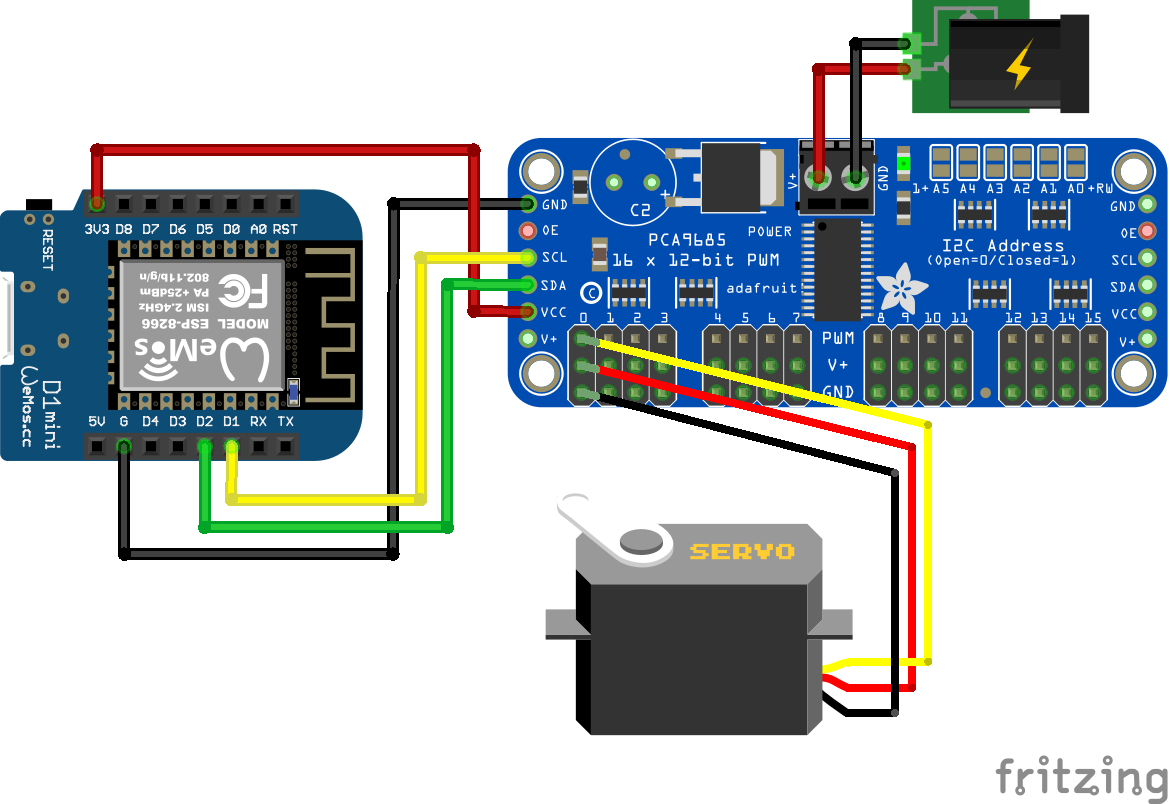Servo PCA9685 External Power Supply ESP8266 WeMos D1 Mini

### How to Parameterize a Servo Motor

Before we can start with the example itself, we have to parameterize our servo motors to find the exact 0 degree and 180 degree position, when the motor is controlled by the PCA9685. Therefore we use the sketch from the first example and have to remember the exact position of the plastic attachment on the motor.

Now we have to find the optimal values for two parameters: MIN_PULSE_WIDTH and MAX_PULSE_WIDTH to define the position of the servo at 0 degree and 180 degrees. The following picture shows the desired result. I found that for the Longruner SG90 Micro Servo Motor 9G RC (KY66-5) the parameters are the following:

• MIN_PULSE_WIDTH = 600
• MAX_PULSE_WIDTH =2600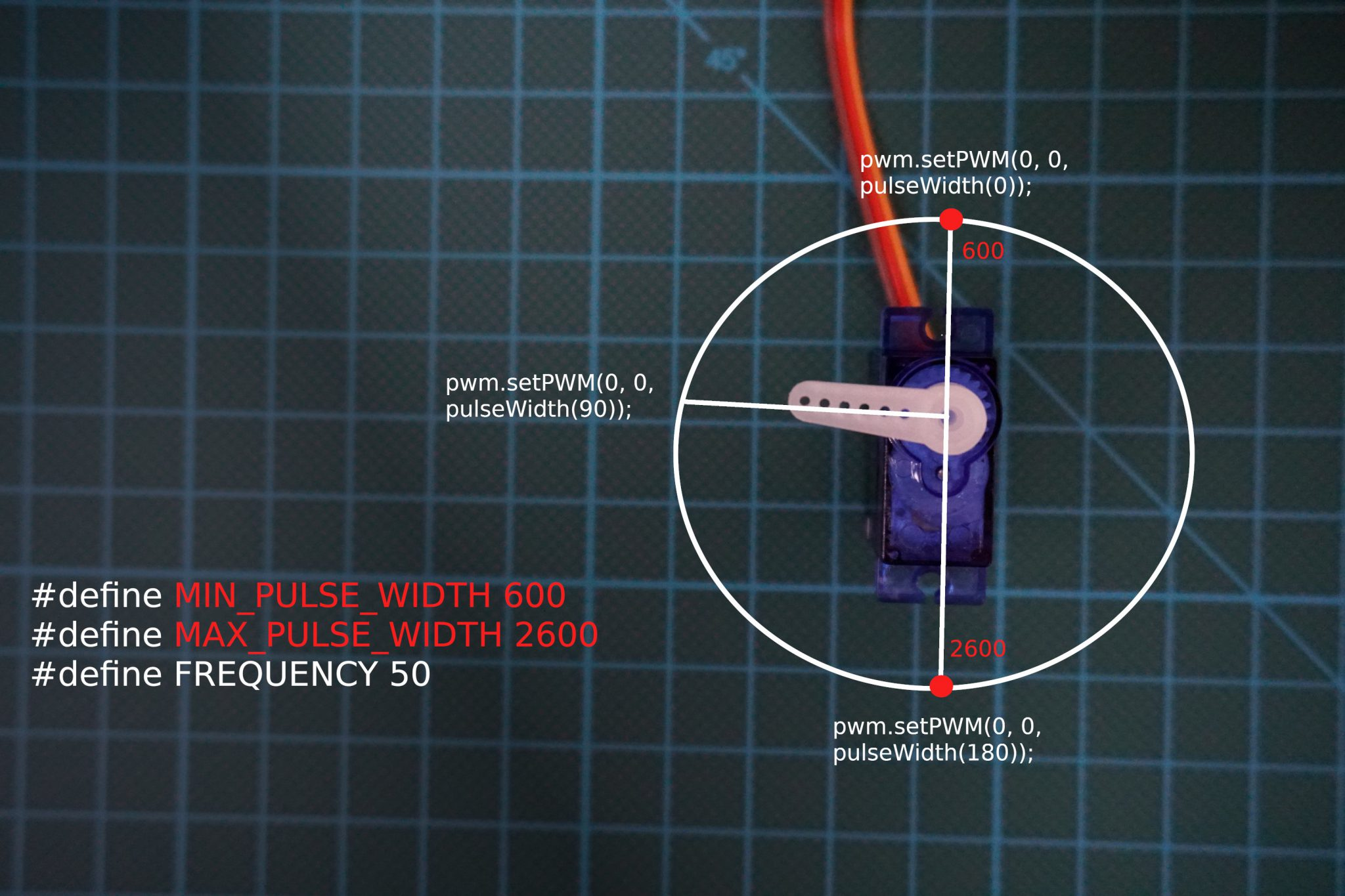Because these values are depending on you individual servos, we now have to find the optimal values for your servo motors.

#### Find value of MIN_PULSE_WIDTH

We use a part of the future full sketch to let the motor settle at 0 degree. Use the following sketch and change the values for MIN_PULSE_WIDTH until the desired position is achieved. Maybe you will overshoot the position, then you have to tweak the parameter again.

The Arduino script might look a little complicated but I explain everything in detail. Also most of the following script is reusable for us for the actual example.

```#include "Wire.h"

#define MIN_PULSE_WIDTH 600
#define MAX_PULSE_WIDTH 2600
#define FREQUENCY 50

void setup() {
pwm.begin();
pwm.setPWMFreq(FREQUENCY);
// find MIN_PULSE_WIDTH: set angle to 0
// find MAX_PULSE_WIDTH: set angle to 180
pwm.setPWM(0,0,pulseWidth(0)); // 0 or 180
}

int pulseWidth(int angle) {
int pulse_wide, analog_value;
pulse_wide = map(angle, 0, 180, MIN_PULSE_WIDTH, MAX_PULSE_WIDTH);
analog_value = int(float(pulse_wide) / 1000000 * FREQUENCY * 4096);
return analog_value;
}

void loop() {
}```

To control the PCA9685 we use the Adafruit_PWMServoDriver library. If you do not know how to install a library you find here a tutorial.

First we include the libraries Wire.h to use the I2C communication and the Adafruit_PWMServoDriver to manage the CA9685 16-Channel Servo Driver board. Then we create an ServoDriver object called pwm with the corresponding I2C HEX address, that is in my case 0x40. To find the right I2C HEX address you can use the I2C HEX code scanner in this article.

Next we define our variables. In this step you need to tweak the parameters MIN_PULSE_WIDTH and set the parameter MAX_PULSE_WIDTH to a fixed value. Nearly all servos use a frequency of 50Hz. You find this information on the data sheet of your motor.

In the setup function the PWM object that we created previously is activated with the frequency of 50Hz. Also we control the servo motor with the setPWM function of the pwm object. The function contains 3 arguments:

1. On which position of the PCA9685 is the servo connected. The numbers are printed on the board starting with 0 up to 15.
2. Constant 0
3. Function to specify the angle: pulseWidth(angle) where angle can be between 0 and 180.
1. To find the optimal value for MIN_PULSE_WIDTH, set the angle to 0
2. To find the optimal value for MAX_PULSE_WIDTH, set the angle to 180

Because we only want to parameterize the servo motor, we need this one iteration from the setup function and have to change the value of MIN_PULSE_WIDTH to get the 0 degree position. Therefore we do not need a loop function.

Now we create the function pulseWidth to calculate the analog value for the motor depending on the desired angle. First the pulse with is calculated between 0 degree and 180 degrees depending on the minimum and maximum pulse width with the map function. Then the analog value for the servo is calculated by dividing the pulse wide by 1,000,000 to convert the value to us per second and multiplied with the frequency and 4096 for a 12 bit resolution. At the end of this function, this analog value is returned.

#### Find value of MAX_PULSE_WIDTH

If you found the right value for MIN_PULSE_WIDTH we do the same with MAX_PULSE_WIDTH  to find the motor position for 180 degrees. We use the same program code and change the value for MAX_PULSE_WIDTH until the 180 degrees position is found. Also you have to change the value for angle in the setPWM function to 180. The value of MIN_PULSE_WIDTH  has to be fixed.

### How to Control Multiple Servo Motors with the PCA9685

In this example we want to use 3 servo motors at the same time. The script has no direct practical use but shows how to control multiple servos in one script over the I2C connection.

```#include "Wire.h"

#define MIN_PULSE_WIDTH 600
#define MAX_PULSE_WIDTH 2600
#define FREQUENCY 50

void setup() {
pwm.begin();
pwm.setPWMFreq(FREQUENCY);
}

int pulseWidth(int angle) {
int pulse_wide, analog_value;
pulse_wide = map(angle, 0, 180, MIN_PULSE_WIDTH, MAX_PULSE_WIDTH);
analog_value = int(float(pulse_wide) / 1000000 * FREQUENCY * 4096);
return analog_value;
}

void loop() {
pwm.setPWM(0, 0, pulseWidth(0));
pwm.setPWM(1, 0, pulseWidth(180));
delay(1000);
pwm.setPWM(4, 0, pulseWidth(0));
delay(1000);
pwm.setPWM(0, 0, pulseWidth(180));
pwm.setPWM(1, 0, pulseWidth(90));
delay(500);
pwm.setPWM(4, 0, pulseWidth(180));
delay(1000);
pwm.setPWM(0, 0, pulseWidth(90));
pwm.setPWM(1, 0, pulseWidth(0));
delay(1000);
}```

Because we know most of the script already from the parametrization, I will concentrate on the changes that we made for this example.

The first part of the script where we include the libraries, create the pwm object and define the variables are the same. The MIN_PULSE_WIDTH and MAX_PULSE_WIDTH are the values from the parametrization.

In the setup function we do not use the setPWM function to turn the motor. This in done in the loop function, where we turn the servo motors in different angles with the argument of the pulseWidth function.

To differentiale the servos, we change the first argument of the setPWM function to the connection pin on the PCA9685 board. In my case, I have a servo connected to pin 0, 1 and 4.

The pulseWidth function is the same compared to the parametrization.

The following video shows the example in action for the Arduino Uno and ESP8266 NodeMCU.### How to use Multiple PCA9685 in a Chain

As said before, it is possible to connect multiple PCA9685 in a chain to connect up to 992 servos with the 2 I2C pins on your microcontroller.
The following fritzing sketch shows you how to connect multiple PCA9685 boards.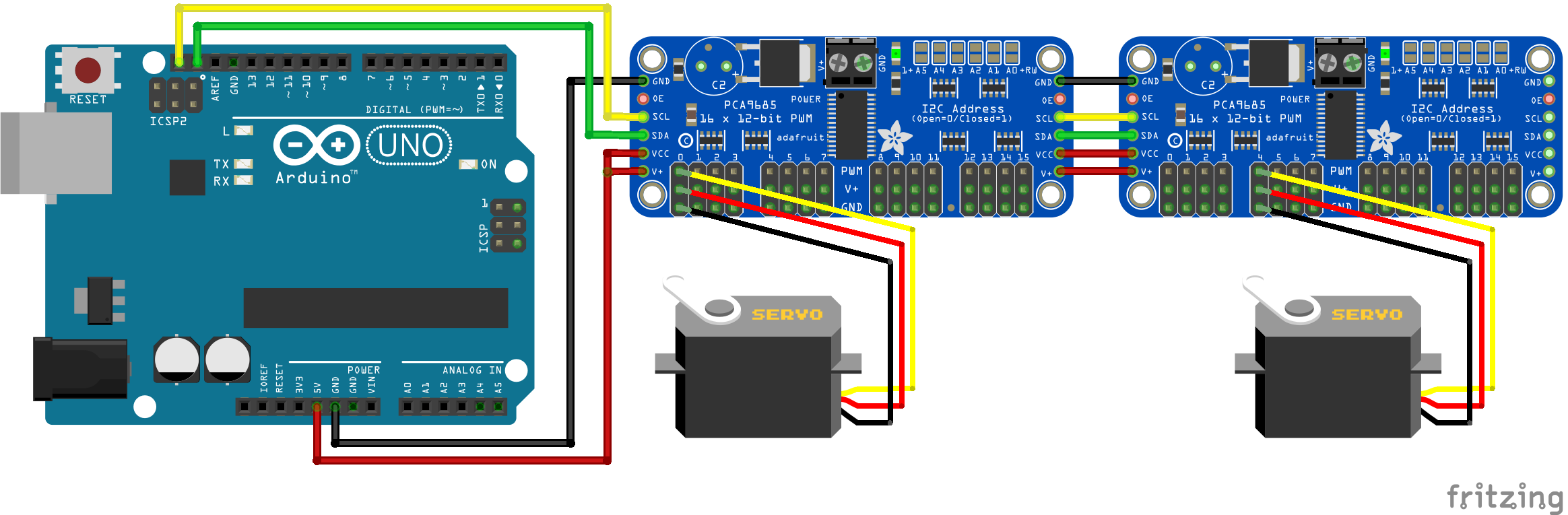You can use the sketch of the previous example and you only have to change the Hex address and create as many ServoDriver objects as you connect PCA9685 boards. The objects could be called pwm1, pwm2, etc. with the individual I2C HEX address. As mentioned before you can use the I2C HEX code scanner from this article to find the right addresses.

Conclusion

In this tutorial we learned a lot about servo motors. First we studied to theory about servos and the functionality. In the second part of the tutorial we got to the practical examples and started with basics examples to turn to motor up to 180 degrees and return to 0 degree. Also we succeeded to turn the servo depending on the position of a potentiometer. After the simple example we learned how to control multiple servos with the PCA9685 and how to connect multiple PCA9685 boards.
If you have any question about the functionality of the servo motor or to any example in this tutorial, please use the comment section below to ask you questions so that I can answer them directly.

### 12 thoughts on “Servo Motor Tutorial for Arduino, ESP8266 and ESP32”

1. Hi
I need help
I’m trying to build a pop-up target with ESP32/esp8266 boards And servo motor and button/switch. This is what I’m trying to build. When the target is hit it will fall and hit the switch and it will restart servo motor to go up 180 degrees Back to 0 Degrees
I’m new at this and I have been working on this for About year. Do you have any idea where I can find a code or diagram For something like this.

Sincerely Buck

• Hi Buck,
I hope that I understand your project right: For example you have a metal plate that is connected to the servo motor. If the plate gets hit, the servo motor should turn for 180 degrees and then go back to the original position.
My first question would be how do you sensor that the target was hit?

• kindly share me your code and diagram because i am also facing same issue as you.

2. What does
>#include // include Servo library
do?

• Hi Ted,
thanks for your comment because this line of code does nothing, and was a mistake on my side. You can include libraries either with #include or #include “Servo.h”. For your Arduino code there is no difference but for my WordPress site the first include syntax is recognized as function and not as text. Therefore on my website the library is not shown in the code box.
I changed the include code for the whole article and now you see what libraries I include in the examples.

3. Think you for reply to my question.
This is What I’m trying to make from (YouTube) homemade air soft gun electric target +tinyplan 97.
But use esp32 and esp8266 .

4. I want to program servos for fingers to move in different positions in a robotic hand. I am using the PCA9685 to run six servos. One servo for the wrist and five for the fingers. Can you do that with the PCA9685? If so, how do you isolate the servos for different movement in the coding. Example: I want the index finger to move just a little and the thumb all the way closed. Can you run servos independently in degrees with the PCA9685?

5. Hi! Great article, i read the other on powering esp32 too.
I need to power esp32 , pca9685 with 3 continuous servo fs90r.
Is there a way to use single battery to power all ?

6. Hallo, toller Artikel.
Ich habe nur eine kurze Frage bezüglich dem Aufbau bzw. Code.
Wäre es möglich zwei Servos mit jeweils einem Poti anzusteuern ohne eine extra PCA Karte?

LG Dennis